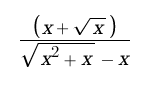# Help me solve this undefined limit please (as x approaches to 0)

#### physicsluver

##### New member
I am trying to find the limit of f(x) as x approaches to 0,
this is an undefined limit that I have to solve using the definition of derivative f'(x).
I have tried to put g(x)=x+√x
and to put h(x)=√(x²+x) - x
but I ended up with another undefined limit:
g'(x)=1+(1/2*√x) which gave me 1(1/0) as x approaches to 0, which equals to infinity.
and
h'(x)=(2x+1)/(2√x²+x) then h'(x)=(1+1/2x)/(1+1/x) which gave me an infinity/infinity as x approaches to 0.ps/ excuse my English. I'm not a native speaker.

#### Dr.Peterson

##### Elite Member
You appear to be using L'Hopital's rule, which applies because the limit has the indeterminate form 0/0; you now have a limit of the form $$\displaystyle \infty/\infty$$, so you could apply L'Hopital's rule again. It is possible, though, that by simplifying this new form, it might not have an indeterminate form. At the least, simplifying might make it easier to apply the rule the second time.

But L'Hopital's rule is not the only way to do it. If you just simplify the function as given, such as by rationalizing the denominator, or merely by dividing numerator and denominator by $$\displaystyle \sqrt{x}$$, you can get it to a form whose limit can be found directly. I would definitely do this rather than L'Hopital's rule!

#### Jomo

##### Elite Member
You do not have the correct h'(x) and there is an error when you tried to simplify it. Try again.